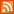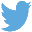meta name="description" content="The Java Script Math object provides the trigonometric functions cosine, sine, tangent, along with many others. All the trigonometric functions return an angular value in radians. Why use radians rather than degrees?" /> Java Script Quick Reference : Java Script Trigonometric MethodsBucaro TecHelpHTTPS Encryption not required because no account numbers orpersonal information is ever requested or accepted by this siteCustom Search# Java Script Trigonometric Methods

The Java Script Math object provides the trigonometric functions cosine, sine, tangent, along with many others. All the trigonometric functions return an angular value in radians. Why use radians rather than degrees?

The 360 degree rotation angle of a circle is totally fabricated by man and is cumbersome to use in mathematical calculations. Radians are in tune with nature as 2 pi radians rotates an arc in a complete circle. Radians make it possible to use algebra to manipulate geometric shapes in Cartesian space (named after the French man Rene Descartes who came up with it while dabbling in, among other things, mathematics). To put it another way, we probably wouldn't have 3D games or computer generated movies without radians.

If you still need to work with degrees, the functions shown below can be used to convert radians to degrees and degrees to radians.

```function rad2deg(radians)
{
degrees = 360 * radians/(2 * Math.PI);
return degrees;
}

{
radians = (2 * Math.PI * degrees)/360;
}
```

As an example, lets use the Math.tan function to solve a common trigonometry problem. We want to determine the height of a structure. We setup a surveyors telescope 100 meters from the base of the structure and rotate the telescope 33.8 degrees to point at the peak of the structure. We can use the basic trigonometric equation shown below.

For our problem we need to rearrange the equation to that shown below.

```height = tan 33.8 degrees * 100;
```

Shown below is the code required to solve the problem.

```var radians = (2 * Math.PI * 33.8)/360;
 .menubtn { margin-top:10px; margin-left:50px; width:220px; opacity:1; border-style:solid; border-color:#009000; background-color:#66ff66; transition-property: opacity; transition-duration: 1s; transition-timing-function: ease-out; } .menubtn:hover { opacity:1; background-color:#e5ff23; } Menu - More Java Script Quick ReferenceRSS FeedFollow @Stephen Bucaro